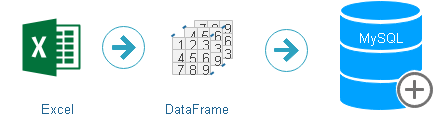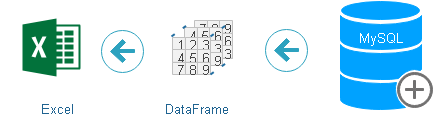# PandasPandas : Python Data analysis tool.

Along with basic understanding of Python, knowledge on these sections are required before learning Pandas.

How to install Python Pandas library
``pip install pandas``
Getting the version of Pandas
``````import pandas as pd
print("Pandas Version : ",pd.__version__)``````
``pip install --upgrade pandas``
Filtering columns and create new DataFrame
``my_new = my_data.filter(['equipment','category'],axis=1)``
OR
``````cols=['MATH','ENGLISH']
my_new=my_data[cols]``````
Displaying columns of the DataFrame
``print(my_new.columns)``
Number of rows in DataFrame.
``print(len(my_new))``
 append() Adding rows list , dictionary to DataFrame Settings Managing DataFrame display option values apply Applying function along an Axis or elements at Get and Set data using rows and columns astype Cast to a specified dtype cut Using segments for categorizing values describe Descriptive statistics of DataFrame or series groupby combining data and aggregate functions get_dummies Convert categorical variable into dummy/indicator variables head First n rows of the DataFrame isnull Checking NaN or None data iloc Values at different position using integer info Detail information about the Dataframe loc Values at different position using column label filter Condition based filtering of rows fillna Filling NaN data merge combining DataFrame and aggregate functions methods Pandas DataFrame methods nlargest n elements in descending sorted values mask conditional replacement of data query Filtering data by using conditions count Number of rows or columns with different options max Max value of required axis mean Mean value of required axis min Min value of required axis shape, size, ndim Dimensions of the DataFrame std Standard Deviation on required axis sum Sum of values of required axis set_index Creating index using one or more columns sort_values Sort columns in ascending or descending tolist From DataFrame to List notnull Not None and Not Null values checking pivot() Reshaped data based on column values pivot_table() Reshaped data with aggregate functions based on column values reset_index Remove index of the DataFrame value_counts counts of unique values unique Unique Data of a column where Data updating based on condition

Five important basic Pandas DataFrame functions info(), head(), tail(), shape,size,ndim, columns

Creating Pandas DataFrame by using Numpy ndarray
validate_email
DataFrame Style
Pandas Date & Time

Exercises
 Exercise1 Basic data handling , DataFrame Exercise1-1 Using cut(), groupby and plotting graphs Exercise-Adv Using groupby and merge of DataFrame Exercise2 Using str.contains(), max(), min(),len() of DataFrame Exercise3 Using date and time functions with groupby of DataFrame Exercise3-2 Using date and time functions of DataFrame Exercise3-3 Using date and time functions with groupby Exercise3-4 Using date and time with where timedelta64

## I/O : Input and output Data from Pandas ( Excel , MySQL , JSon)We can’t store data in Pandas DataFrame. We can process the data by using Pandas DataFrame after reading data from different sources. Similarly after processing we can save data in different files or database by using available tools.

Filtering records
 loc Values at different position using column label rows Filtering rows based on data

## handling string using str methods

 str.contains string matching against data columns str.contains.sum Max Min Sum of any column Convert Case Lower to Upper and vice versa split() Breaking string using delimiter slice() Substring by breaking string cat() Concatenate strings count() Number of occurrences of pattern replace() Replace part of string by regex len() Length of the data in our DataFrame
Plotting Graphs using Data
 Plotting graphs Creating different type of graphs using DataFrame MySQL data Creating different type of graphs MySQL data Exercise Graph using data from Excel file
Managing Date
 Pandas Date and time Managing Date and time in Pandas DataFrame

## Excel to MySQL``````import pandas as pd
# reading data from root of D drive.
from sqlalchemy import create_engine

### Creating new table emp or appending existing table
my_data.to_sql(con=engine,name='emp',if_exists='append')``````

## MySQL to Excel``````import pandas as pd

from sqlalchemy import create_engine

sql="SELECT * FROM emp "
my_data = pd.read_sql(sql,engine )

my_data.to_excel('D:\emp2.xlsx')``````

## Columns and rows

 columns List of column headers of a DataFrame rename rename columns of DataFrame add_suffix adding suffix to column names of a DataFrame add_prefix adding prefix to column names of a DataFrame drop Delete columns or rows

## Data Cleaning

Various methods in Pandas to clean data. Read from different sources then remove or fill with matching data in rows and columns

Get the list of all functions of Pandas. ( Used dir() )
``````import pandas as pd
print(len(dir(pd))) # 139``````
``````for i in dir(pd):
print(i)``````

## Parameters of functions

There are some common parameters used in Pandas functions. Understanding them will help in quick learning of functionality.
 inplace Boolean ( True / False ), Result is written back to same dataframe ( True ). The source dataframe is changed. False otherwise.

Upload Excel or CSV file in google drive and connect from Colab Python platform using mount drive

Subscribe to our YouTube Channel here

## Subscribe

* indicates required
Subscribe to plus2netplus2net.com

Post your comments , suggestion , error , requirements etc here